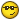###Author Topic: How is energy production calculated from light level and # of chloroplasts?  (Read 1550 times)

####PhiNotPi

• Bot Builder
•• Posts: 64##### How is energy production calculated from light level and # of chloroplasts?
« on: February 13, 2014, 09:43:19 PM »
From what I have read, the energy production of a single veggie is determined by the number of chloroplasts and the light level (which is itself dependent on the density of robots on screen, etc.).

I've done a lot of digging, and I haven't been able to find the formula which relates light level, # of chloroplasts, and energy production.  I managed to find this image, but I want to know the underlying formulas which created those lines on the graph.

I'm trying to write a "smart veggie" which determines how many chloroplasts it should produce for a given light level.  If anyone knows the math formula which calculates energy production, that would be greatly helpful.
I am biased neither towards nor against any single mathematical constant.

####Botsareus##### Re: How is energy production calculated from light level and # of chloroplasts?
« Reply #1 on: February 14, 2014, 10:15:19 AM »
Got excel?

I usually just use:

Code: [Select]
cond 'new gene from Botsareus and Panda  *.chlr  *.light  <start
I does not make sense to reduce chloroplasts in most of the cases because of the cost for creating them.

####PhiNotPi

• Bot Builder
•• Posts: 64##### Re: How is energy production calculated from light level and # of chloroplasts?
« Reply #2 on: February 14, 2014, 01:13:30 PM »
Thanks.

I combined those formulas into one large formula, and I assumed this was how light was calculated.

Given a light level L (0-32000), a chloroplast count C (0-32000), and a feeding rate R, then the following formula gives the amount of energy.  Please pardon the horrible math formatting.

Some things of interest:

The following gives the maximum sustainable number of chloroplasts at a given light level.  This means that any larger number of chloroplasts will result in a negative total feeding rate.  Notice how this does not depend on the feeding rate R.

Likewise, the inverse formula gives the "break-even point" for a given number of chloroplasts.  Any less light, and the chloroplasts would have net losses.

Using this, I calculated that a light level of at least 9841 can sustain any number of chloroplasts.

It is also interesting to look at the optimal number of chloroplasts at a given light level, which is given by the following.  Any larger or smaller number of chloroplasts would result in less than the maximum possible energy.

Likewise, the inverse formula gives the light level for which a given number of chloroplasts is the optimal number.  This may not be as useful.

Once the light level reaches reaches the critical value of 17449, then the optimal number of chloroplasts is greater than 32000.  This means that "more is always better" in terms of how many chloroplasts the bot should have.  Only once the light level falls below 17449 does a robot have to worry about computing the optimal number of chloroplasts.  Also, the robot only has to worry about energy losses once the light level falls below 9841.

Edit 1:

If the light level is below 14987, then the actual feeding rate of the robot (with an optimal # of chloroplasts) is strictly less than the value of R.  This is the light level at which the best possible feeding rate is equal to the value entered into the settings, assuming that "nrg per veggie per cycle" is selected.  Also, assuming the best possible environmental conditions (max light and max chloroplasts), then the actual feeding rate can be no greater than .
« Last Edit: February 14, 2014, 03:56:59 PM by PhiNotPi »
I am biased neither towards nor against any single mathematical constant.

####Botsareus##### Re: How is energy production calculated from light level and # of chloroplasts?
« Reply #3 on: February 14, 2014, 03:04:43 PM »
Brilliant